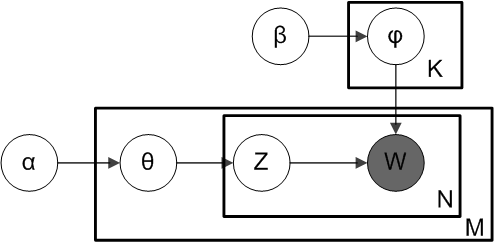# LDA主题模型学习心得

## LDA主题模型

### LDA 简介

LDA模型：Latent Dirichlet Allocation是Blei 等人于2003年提出的基于概率模型的主题模型算法，它是一种非监督机器学习技术，可以用来识别大规模文档集或预料库中的潜在隐藏的主题信息。
LDA算法的核心思想：每篇文章由多个主题mix混合而成的，而每个主题可以由多个词的概率表征。该方法假设每个词是由背后的一个潜在隐藏的主题中抽取的。

1.对于每篇文档，从主题分布中抽取一个主题。
2.从上述抽到的主题所对应的单词分布中抽取一个单词。
3.重复上述过程直至遍历文档中的每个单词。

### LDA 算法的输入

1.每篇文章的各个词被指定的主题编号:tassign-model.txt
2.每篇文章的主题概率分布θ$\theta$:theta-model.txt
3.每个主题下的词概率分布ϕ$\phi$:phi-model.txt
4.程序中词语word的id映射表：wordmap.txt
5.每个主题下ϕ$\phi$概率排序从高到低top n特征词：twords.txt

### 必备的数学知识

#### Gamma函数

gamma函数其实就是阶乘的函数，比如n!=1*2*3*….n,这个阶乘形式可以更一般化，不局限于整数。而更一般的函数形式就是gamma函数：

Γ(x)=+0ettx1dt(x>0)

Γ(1)=+0etdt=[et]+0=1$\Gamma(1)=\int_0^{+\infty}e^{-t}dt=-[e^{-t}]_0^{+\infty}=1$,Γ(12)=π$\Gamma(\frac{1}{2})=\sqrt{\pi}$,Γ(x+1)=xΓ(x)$\Gamma(x+1)=x\Gamma(x)$,Γ(n)=(n1)!$\Gamma(n)=(n-1)!$

P=Cknpk(1p)nk

#### beta分布（beta distribution）

beta分布是指一组定义在区间(0,1)的连续概率分布，有两个参数α$\alpha$β$\beta$,且α$\alpha$,β$\beta$>0.它是一个作为伯努利分布与二项分布的共轭先验分布的密度函数。

Beta分布的概率密度函数：

f(x;α,β)=1B(α,β)xα1(1x)β1

B函数与Gamma函数的关系：
B（α,β$\alpha,\beta$）= Γ(α)Γ(β)Γ(α+β)$\frac{\varGamma(\alpha)\varGamma(\beta)}{\varGamma(\alpha+\beta)}$
Beta分布的期望可以用αα+β$\frac{\alpha}{\alpha+\beta}$来估计。

#### 多项分布（multinomial distribution）

f(x1,...,xk;n,p1,...pk)=Γ(ixi+1)iΓ(xi+1)i=1kpxii

### LDA的Gibbs Sampling推导

#### unigram假设

unigram假设其实简单的理解就是词袋模型

#### Latent Dirichlet ALLocation IntroM代表训练语料中的文章数；
K代表设置的主题数；
V代表训练语料库中出现的所有词的词表；
θ$\theta$是一个M*K的矩阵，θ⃗ m$\vec{\theta}_m$代表第m篇文章的主体分布；
ϕ$\phi$是一个K*V的矩阵，ϕ⃗ k$\vec{\phi}_k$代表编号为k的主题之上的词分布；
α$\alpha$是每篇文档的主题分布的先验分布Dirichlet分布的参数（超参数），其中θ⃗ i$\vec{\theta}_i$~Dir(α⃗ )$Dir(\vec{\alpha})$;
β$\beta$是每个主题的词分布的先验分布Dirichlet分布的参数（超参数），其中ϕk$\phi_k$~Dir(β⃗ )$Dir(\vec{\beta})$;
W是可被观测的词；

1.选择θ⃗ i$\vec{\theta}_i$~Dir(α⃗ )$Dir(\vec{\alpha})$;(i=1,2,…,M)
2.选择ϕk$\phi_k$~Dir(β⃗ )$Dir(\vec{\beta})$;(k=1,2,…,K)
3.对于每个单词位置Wi,j$W_{i,j}$(j=1,…,Ni$N_i$),(i=1,…,M)
4.选择一个topic主题服从zi,j$z_{i,j}$~Multinomial(θi)$Multinomial(\theta_i)$
5.选择一个word词服从wi,j$w_{i,j}$~Multinomial(ϕzi,j)$Multinomial(\phi_{z_{i,j}})$

i.从服从θm$\vec{\theta_m}$~Dir(α⃗ )$Dir(\vec{\alpha})$的箱子中抽取一个文档–主题骰子，重复一下过程
ii.投掷这个doc-topic骰子，得到一个topic编号z
iii.从服从ϕk$\phi_k$~Dir(β⃗ )$Dir(\vec{\beta})$分布的箱子里共有主题–单词骰子中选择编号为z的那个，投掷这个骰子，于是得到一个词。

#### 采样公式:Collapsed Gibbs Sampling采样

p(w⃗ ,z⃗ |α⃗ ,β⃗ )=p(w⃗ |z⃗ ,β⃗ )p(z⃗ |α⃗ )

#### 总结

bH(q)=b1NNi=1logbq(xi)

perplexity的值越小，说明模型越好。11-13

#### gensim包LDA主题分析，并输出每条矩阵属于每个主题的概率

05-21

07-115217

#### Python LDA主题模型实战

09-11264

#### LDA学习心得

09-299894

#### LDA主题模型学习总结

06-069244

#### LDA主题模型学习笔记1：模型建立

09-293736

#### gensim实现LDA(Latent Dirichlet Allocation)算法提取主题词(topic)

06-081万+

#### lda主题模型python实现篇

11-121226

#### 基于《机器学习》学习整理- chapter 3

12-242万+

#### 主题模型TopicModel：LDA参数推导、选择及注意事项

08-143292

#### LDA模型详解

08-012866

#### 最新微信小程序源码

09-0323万+

#### 微信小程序源码-合集1

07-313万+

#### 【sklearn】利用sklearn训练LDA主题模型及调参详解

04-114816

#### 主题提取LDA方法

10-221311

#### LDA主题模型浅析 -- 笔记

04-251380

#### 彻底理解文本主题模型LDA(极致原理讲解+实战)

11-08706

#### 基于《机器学习》学习整理- chapter 1©️2019 CSDN 皮肤主题: 大白 设计师: CSDN官方博客点击重新获取扫码支付